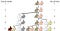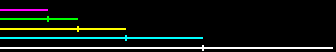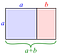# Fibonacci Everywhere!

I first learned about this interesting sequence when I was playing around with forex trading (which is trading different currencies) and learned that the fibonacci sequence is a technical analysis tool used in conjunction with other tools used by professional traders to calculate the forecast of a potential end of a correction by taking a percentage of the previous move.

As you can see I did not know how to properly apply this analytical tool and missed out on becoming filthy rich with trading currencies and now I have to learn to become a programmer to make living in the future…

# So who was Fibonacci?

The fibonacci numbers are named after Leonardo Fibonacci of Pisa, Italy, who actually did not discover the sequence which had been described in Indian mathematics long ago. It was Leonardo Fibonacci who introduced the sequence to Western European mathematic s through the used in his important book ”Liber Abaci” (Book of Calculation) in 1202 were he used the sequence trying to explain the population of rabbits.Leonardo considers a hypothetical situation where there is a pair of rabbits put in the field. They mate at the end of one month and by the end of the second month the female produces another pair. The rabbits never die, mate exactly after a month and the females always produce a pair (one male, one female). The puzzle that Fibonacci posed was: how many pairs will there be in one year? If one calculates then one will find that the number of pairs at the end of nth month would be Fn or the nth Fibonacci number. Thus the number of rabbit pairs after 12 months would be F12 or 144.

# Fibonacci and the Golden Ration

As you might already know the sequence starts with 1 and 1, after that, each new number is the product of adding the previous two numbers. So you end up with

1, 1, 2, 3, 5, 8, 13, 21, 34, 55, 89, 144…

If you divide the numbers for example 144/89 you end up with a number of 1.618 which is considered to be the “Golden Number or Golden Ratio”

The proportion is also said to be aesthetically pleasing due to which several artists and architects, including Salvador Dali and Le Corbusier, have proportioned their work close to the golden ratio. The Fibonacci sequence and the golden ratio are intimately interconnected. The ratio of consecutive Fibonacci numbers converges and approaches the golden ratio and the closed-form expression for the Fibonacci sequence involves the golden ratio.Each line is 1.618… times longer than the one before it. (Conversely, a section drawn at 0.618 (or 61.8%) of each line equals the length of the one before it.)A golden rectangle (in pink) with longer side a and shorter side b, when placed adjacent to a square with sides of length a, will produce a similargolden rectangle with longer side a + band shorter side a. This illustrates the relationship {\displaystyle {\frac {a+b}{a}}={\frac {a}{b}}\equiv \varphi }

A Fibonacci spiral is a series of connected quarter-circles drawn inside an array of squares with Fibonacci numbers for dimensions. The squares fit perfectly together because of the nature of the sequence, where the next number is equal to the sum of the two before it. Any two successive Fibonacci numbers have a ratio very close to the Golden Ratio, which is roughly 1.618034. The larger the pair of Fibonacci numbers, the closer the approximation. The spiral and resulting rectangle are known as the Golden Rectangle.

# Why I think this sequence is so fascinating.

The Fibonacci numbers describe a variety of phenomena in art, music, and nature. The numbers of spirals on most pinecones and pineapples are Fibonacci numbers. The arrangement of leaves or branches on the stems of many plants are Fibonacci numbers. On a piano, the number of white (8) keys and black (5) keys in each octave (13) are all Fibonacci numbers. The lengths and widths of many rectangular objects such as index cards, windows, playing cards, and light-switch plates are consecutive Fibonacci numbers.

# Fibonacci in Nature

Sunflowers are excellent examples of the Fibonacci sequence because the seed heads in the centre of the flower are organized in two sets of spirals — short ones running clockwise from the centre, and longer ones running counter-clockwise. If you count the spirals in a consistent manner, apparently you will always find a Fibonacci number.

The Fibonacci sequence can also be seen in the way tree branches form or split. A main trunk will grow until it produces a branch, which creates two growth points. Then, one of the new stems branches into two, while the other one lies dormant. This pattern of branching is repeated for each of the new stems. A good example is the sneezewort. Root systems and even algae exhibit this pattern.

Here are a couple more examples where you could find the fibonacci spiral in nature.

Not surprisingly, spiral galaxies also follow the familiar Fibonacci pattern. The Milky Way has several spiral arms, each of them a logarithmic spiral of about 12 degrees.

# Fibonacci in the human body

There are many examples but let me use the hand and in particular the finger to demonstrate the accurance of fibunacci.

Each section of your index finger, from the tip to the base of the wrist, is larger than the preceding one by about the Fibonacci ratio of 1.618, also fitting the Fibonacci numbers 2, 3, 5 and 8

# Fibonacci in Photography and art

In photography the phi grid is the interpolation of the fib spiral and is these days, considered to be a fundamental technique to establish a pleasing composition in a frame. The goal is to align your subject with the lines created by the spiral or use it as a kind of separator to create the right feel for a frame.

As you can see there are plenty of examples where the fibunacci sequence appears all around us and we don’t pay attention to this mathematical miracle that feels like a mysterious quotient bringing a universal form of harmony to elements of nature mathematic musics art.

Also I found the single reason why Donald Trump was elected president…

So never underestimate the hidden powers of the Fibonacci sequence.

Sources